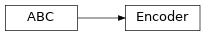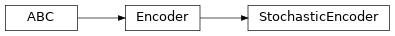# garage.np.embeddings¶

Embedding encoders and decoders which use NumPy as a numerical backend.

class Encoder[source]

Bases: abc.ABCBase class of context encoders for training meta-RL algorithms.

property spec(self)

garage.InOutSpec: Input and output space.

property input_dim(self)

int: Dimension of the encoder input.

property output_dim(self)

int: Dimension of the encoder output (embedding).

reset(self, do_resets=None)[source]

Reset the encoder.

This is effective only to recurrent encoder. do_resets is effective only to vectoried encoder.

For a vectorized encoder, do_resets is an array of boolean indicating which internal states to be reset. The length of do_resets should be equal to the length of inputs.

Parameters

do_resets (numpy.ndarray) – Bool array indicating which states to be reset.

class StochasticEncoder[source]An stochastic context encoders.

An stochastic encoder maps an input to a distribution, but not a deterministic vector.

property distribution(self)

object: Embedding distribution.

property spec(self)

garage.InOutSpec: Input and output space.

property input_dim(self)

int: Dimension of the encoder input.

property output_dim(self)

int: Dimension of the encoder output (embedding).

reset(self, do_resets=None)

Reset the encoder.

This is effective only to recurrent encoder. do_resets is effective only to vectoried encoder.

For a vectorized encoder, do_resets is an array of boolean indicating which internal states to be reset. The length of do_resets should be equal to the length of inputs.

Parameters

do_resets (numpy.ndarray) – Bool array indicating which states to be reset.